Click to Chat

1800-1023-196

+91-120-4616500

CART 0

• 0

MY CART (5)

Use Coupon: CART20 and get 20% off on all online Study Material

ITEM
DETAILS
MRP
DISCOUNT
FINAL PRICE
Total Price: Rs.

There are no items in this cart.
Continue ShoppingParallel and Sequential Reactions

Table of Content

Parallel or Competing Reaction

Example of Parallel Reactions

Consecutive or Sequential  Reactions

Example of Sequential Reactions

Related Resources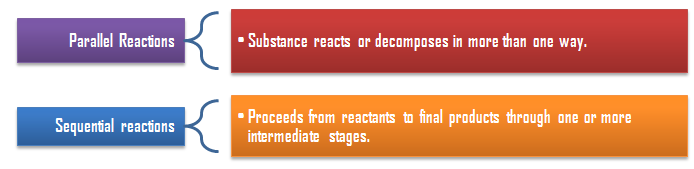Parallel or Competing ReactionThe reactions in which a substance reacts or decomposes in more than one way are called parallel or side reactions.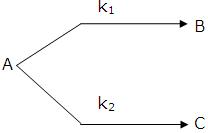If we assume that both  of them are first order, we get.

k1 = fractional yield of B × kav

k2 = fractional yield of C × kav

If k1 >  k2 then

A → B main and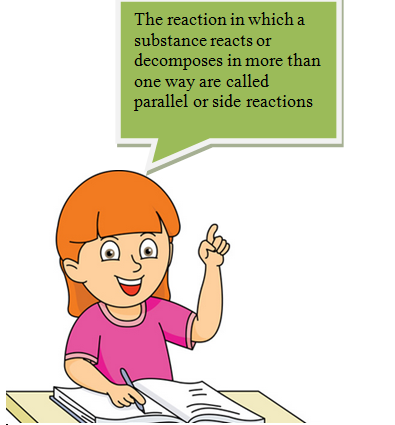A → C is side reaction

Let after a definite interval x mol/litre of B and y mol/litre of C are formed.

i.e

This means that irrespective of how much time is elapsed, the ratio of concentration of B to that  of C from the start (assuming no B  and C in the beginning ) is a constant equal to k1/k2.Refer to the following video for parallel reactions:

Example of Parallel Reactions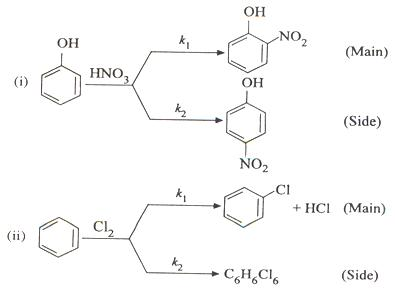Consecutive or Sequential  Reactions

This reaction is defined as that reaction which proceeds from reactants to final products through one or more intermediate stages. The overall reaction is a result of several successive or consecutive steps.

A → B → C and so on

Example of Sequential Reactions

Decomposition of ethylene oxide

(CH2)2O  CH3CHO

CH3CHO  CO + CH4

The pyrolysis of acetone

(CH3)2CO  CH4 + CH2 =C=O

CH2 =C=O  C2H4 + COFor the reaction

…....(i)

…......(ii)

….......(iii)

Integrating equation (i), we get

Now we shall integrate equation (ii) and find the concentration of B related to time t.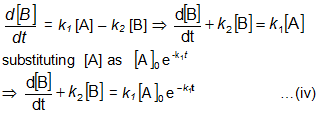Integration of the above equation is not possible as we are not able to separate the two variables, [B] and t. Therefore we multiply equation (4) by an integrating factor , on both the sides of the equation.Integrating with in the limits 0 to t.Now in order to find [C], substitute equation (vi) in equation (iii), we get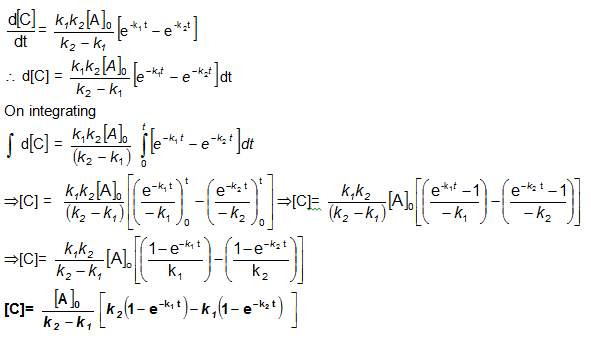Bmax and tmax:

We can also attempt to find the time when [B] becomes maximum. For this we differentiate equation (vi)  and   find d[B]/dt & equate it to zero.Substituting  equation (vii)  in equation (vi)Question 1:

Parallel  reactions take place in

a. more than one way

b. more than one step

c. in one way but more than one step

d. in one step but more than one way

Question 2:

Sequential reactions have

a. more than one way to proceed toward product

b. more than one step

c. no intermediate

d. catalyst

Question 3:

Which one of the following statements is correct?

a. parallel reactions are also known as consecutive reactions

b. Competingreactions  have more than one step

c. Sequential reactions are also called consecutive reactions

d. Consecutive reactions do not have intermediates.

Question 4:

Decomposition of ethylene oxide is

a. parallel reaction

b. competing reaction

c.both parallel as well as competing reaction

d. sequential reactionQ.1
Q.2
Q.3
Q.4

a
b
c
d

Related Resources

Refer to the Books of Chemistry for IIT JEE

You can also  refer Zero Order Reaction

To read more, Buy study materials of Chemical Kinetics comprising study notes, revision notes, video lectures, previous year solved questions etc. Also browse for more study materials on Chemistry here.### Course Features

• 731 Video Lectures
• Revision Notes
• Previous Year Papers
• Mind Map
• Study Planner
• NCERT Solutions
• Discussion Forum
• Test paper with Video Solution